# Acoustic radiation features and structural-acoustic sensitivity of channel beam

Acoustic radiation features and structural-acoustic sensitivity of channel beam

Jinbo Song Rui Song Zhi Xiong

Luqiao Department, Jiangxi Vocational and Technical College of Communication, Nanchang 330013, China

Nanchang Institute of Technology Jiangxi Nanchang, Nanchang 330013, China

Corresponding Author Email:
jxjtxylqx@126.com
Page:
35-45
|
DOI:
https://doi.org/10.3166/TS.35.35-45
|
Accepted:
|
Published:
30 April 2018
| Citation

OPEN ACCESS

Abstract:

This paper aims to disclose the effects of structural parameters on the structural noise of channel beam. To this end, a finite-element model of rail transit channel beam was established based on vehicle-track coupling dynamics, and the vibration response of the channel beam was computed under the load of train. Then, the noise features of the rail transit channel beam were analyzed by indirect boundary element method, and the structural-acoustic sensitivity of the channel beam was calculated by finite-difference method. After that, the author discussed how the bottom plate thickness, web thickness and flange plate thickness of the channel beam affect the linear sound pressure level at different frequencies and different points of the acoustic field. The results show that the peak noise frequency of the rail transit channel beam fell in the range of 31.5~80Hz. The thickening of the bottom plate helps to control the structural noise in a small near-bridge area but cannot reduce the noise at far-field points. The thickening of the web will increase the peak sound pressure level at near-field points and reduce the noise at far-field points. The bottom plate thickness and web thickness have a small impact on the sound pressure of the field points, so does the effect of flange plate thickness on the structural noise of the channel beam. The research findings provide a reference for noise reduction of bridge structures and the optimization of bridge design.

Keywords:

channel beam, indirect boundary element, structural noise, structural-acoustic sensitivity

1. Introduction

As urbanization accelerates in China, traffic congestion becomes increasingly serious. Rail transit is a solution to this problem. The web of channel beams in rail transit systems can effectively block wheel-rail noises and reduce the average structural height. Therefore, channel beams are used in more and more urban rail transits.

Considering that the structural noise produced by the channel beam has seriously restricted its development, we think it necessary to study on the vibration and noise reduction measures of the channel beam.

At present, domestic and foreign scholars have conducted quantitative research on the noise of bridge structure. For the interaction of dynamics between vehicles, tracks and bridges,

Li et al. (2015) used boundary element method (BEM) to study the noise of vehicle-track-bridge coupling and boundary element in frequency domain for high-speed concrete box girder. Zhang et al. (2015) used the FE-BEM method to study the acoustic pressure distribution around a bridge for a noisy highway steel bridge, and analyzed the effects of vehicle speed and road irregularity on the noise. Wu and Liu, (2012) combined the power flow method in the frequency domain and the frequency domain boundary element technology to compare the noise characteristics of U-shaped beams and box girders on the elevated line of Shanghai rail transit. Li et al., (2012) studied using 3 d boundary element beam structure vibration and noise, the simulation results and the measured values are in good agreement, and points out that the bridge structure noise has strong directivity. Janssens and Thompson, (1996) use the statistical energy method (SEA) to predict railway bridge noise and compare the different types of Bridges. Bewes et al., (2006) with a steel and concrete composite bridge as the research object, to calculate the power input to the bridge is calculated using statistical energy method (SEA) and structure noise.

Stuber, (1975) has tested the noise of railway steel Bridges with different rail structure. The test results show that the different orbital structures have great influence on the bridge noise. Ngai and Ng, (2002) has tested the vibration and noise of a concrete viaduct in the west rail of Hong Kong. The test results show that the vibration and noise of the box beam are in the 20-157Hz. Based on different theoretical methods and field measurements, Zhang el al., (2015) systematically studied the railway concrete box girders and studied the root causes of peak noise in the inner and outer cavity. Based on vehicle track bridge coupled vibration analysis and frequency domain boundary element method, Li et al., (2013) studied the noise of concrete box girder of high-speed railway by boundary element method.

The structural-acoustic sensitivity is to study how greatly the acoustic evaluation index values can change with the change of structural design variables or parameters. The sensitivity analysis can help engineers find the principal parameters of structural modification, so as to improve the design in efficiency and quality and reduce the design cost. Based on the theories of vehicle-track coupled dynamics (Lei, 2015; Zhai, 2007), the acoustic radiation characteristics of the vehicle-loaded channel beam are analyzed in this paper by using the approaches of finite element and boundary element. Finally, be means of finite difference method, the structural-acoustic sensitivity of the channel beam is calculated. The influence of the floor slab thickness, web thickness and the thicknesses of left and right flanges on the sound pressure levels at field points is analyzed, with frequency as the variable. The analysis results provide some references for the acoustic optimization of channel beam structures.

2. Noise prediction of channel beam structure

2.1. Establishing the finite element model

We selected a rail transit channel beam to establish the finite element model, whose parameters are: standard span of 30m, roadbed width of 3.634m, and floor slab thickness of 0.24m. The most prominent feature of this channel beam is the specially designed arched web at the mere thickness of 0.24 m. The contact surface of the web and the roadbed plate is smoothed, as shown in Figure 1. The fully prestressed beam has reached the concrete strength grade of C50, and the rail bearing stand and the bridge deck slab are cast as a whole.

## 1.png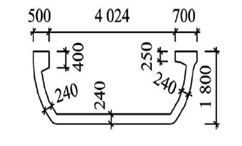Figure 1. Standard cross-section form of the channel beam (unit: mm)

In the finite element model, the rail, fastener and rail bearing stand are simulated by beam188 (beam), combine14 (spring), and solid185 (solid element), respectively. We use shell63 (shell element with some thickness) to simulate the bridge, because the global and local vibration characteristics and parameter sensitivity can be well reflected by shell elements. As the rail bearing stand and the bridge deck slab are cast as a whole, the channel beam and the rail bearing stand are consolidated by node coupling. The finite element model is shown in Figure. 2.

## 2.png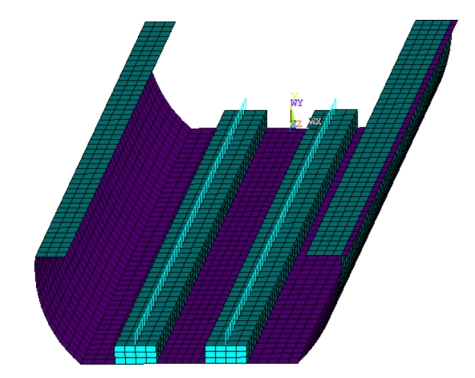Figure 2. Finite element model of channel beam

2.2. Vehicle-track coupled wheel/rail force

Loads are applied to the specimens of two Type A subways (for different types of urban rail cars, please refer to Engineering Construction Standards of Urban Rail Transit in the People’s Republic of China), and the operation speed is set as 89Km/h. Track irregularity samples are obtained according to the track irregularity spectra provided in document. Considering the spectrum range is 0.1-25m, we select the longitudinal irregularity samples and show them in Figure 3. After calculation, the irregularity excitation frequency fluctuates between 0.89Hz and 222.2Hz, which can meet the analysis requirements of the global and local vibration characteristics and the structural noises of the channel beam. The metro train operation process is simplified as a series of time-varying concentrated forces, which is then applied to the finite element model of the channel beam (Chen et al., 2007) to calculate beam vibration. The step length of the loading time is 0.0018s

## 3.pngFigure 3. Longitudinal irregularity samples

2.3. Indirect boundary element method for structural noise of channel beam

Compared to the finite element method, the boundary element method reduces the computational dimension of structural noise and automatically satisfies the far-field radiation condition at the boundary. When calculating the radiation noises generated from the structural vibration of the channel beam that serves for rail transit, the boundary grids of the corresponding boundary element are not closed. Therefore, the boundary integral equation should be solved by means of indirect boundary element, which can be deduced from the direct boundary element method.

Based on the conservation law of fluid media and some basic assumptions about sound waves, we have the following Helmholtz equation:

$\left(\nabla^{2}+k^{2}\right) p=0$  (1)

where k = ω / c, p is the sound pressure; k is the wave number ω is the angular frequency c is the sound velocity in the medium. The surface of the channel beam can be seen as the impervious boundary of small amplitude motion, which satisfies the Neumann boundary condition below.

$\partial p / \partial n=i \omega \rho v$        (2)

where n is the normal vector outside of the boundary of the channel beam surface; v is the normal vector of the vibration velocity in the boundary surface; ρ is the density of the fluid. The structural noise radiation of the channel beam is not present at the infinite far sound field, and thus it has to satisfy the Sommerfield condition:

$\lim _{r \rightarrow \infty} \int_{\Gamma}(\partial p / \partial r+i k p) d S_{\Gamma}=0$   (3)

where pis the sound pressure vector.r is the distance of the field point in the sound field from the source point. Γ is the wave front that is r far from the source point. ЅΓ is the area of the wave front. With the weighted residual method, the basic solution of the free space Green’s function G (rp, rQ) in equation (1), and some boundary conditions, we can deduce the Helmholtz boundary integral function

$\int_{H}\left(G\left(r_{P}, r_{Q}\right) \frac{\partial G\left(r_{P}, r_{Q}\right)}{\partial n}\right) d s_{Q}=\left\{\begin{array}{ll}{p\left(r_{P}\right)} & {P \in E} \\ {\frac{1}{2} p\left(r_{P}\right)} & {P \in H} \\ {0} & {P \in I}\end{array}\right.$      (4)

where G(rp, rQ)=е–ik|rp–rQ|/(4π|rp rQ|)

In this equation, rp is the position vector of the field point P in the sound field, rQ is the position vector of the source point of the boundary element model, and p(rp) is the sound pressure at the field point P; E, H and I represent the positions of “outside the boundary”, “on the boundary” and “in the boundary”, respectively. SQ is the boundary element area at the source point Q. Different from direct boundary elements, the indirect boundary element method includes the concept of potential. As the sound field covers both sides of the boundary element grid, it is necessary to calculate the sound pressure (double-layer potential) difference and sound pressure gradient (single-layer potential) difference on both sides of the boundary element. On each side, Equation (4) has a result. The difference of the two results is just the sound pressure at an arbitrary field point P.

$p\left(r_{p}\right)=\int_{H}\left(G\left(r_{p}, r_{Q}\right)-\Delta p\left(r_{Q}\right) \partial\left(r_{p}, r_{Q}\right) / \partial n\right) d s_{Q}$

where

$\left\{\begin{array}{l}{\delta\left(r_{Q}\right)=-i \omega \rho\left(v\left(r_{Q 1}\right)-v\left(r_{Q 2}\right)\right)} \\ {\Delta p\left(r_{Q}\right)=p\left(r_{Q 1}\right)-p\left(r_{Q 2}\right)}\end{array}\right.$       (5)

In the formula, δ(rQ) and Δp(rQ) are the respective sound pressure gradient difference and sound pressure difference on both sides of the boundary element Q. In terms of the indirect boundary element method, we can identify unknown nodes of single/double-layer potential by using the following equation:

$\left(\begin{array}{cc}{B} & {C} \\ {C^{T}} & {D}\end{array}\right)\left(\begin{array}{c}{\delta} \\ {\Delta p}\end{array}\right)=\left(\begin{array}{c}{f \sigma} \\ {f \mu}\end{array}\right)$     (6)

where δ and Δp are the vectors of double-layer potential and single-layer potential, respectively; B, C and D are the non-singular complex matrices that are related to the structure’s surface shape and interpolating shape function; ƒδ and ƒΔp are excitation vectors that are influenced by interpolating shape function but are decided by the normal velocity speed of the structure surface. The double-layer potential and single-layer potential of different nodes in the boundary element method are determined. The sound pressure of an arbitrary point in the sound field is

$p(r)=A_{\delta}^{T} \delta+A_{\Delta p}^{T} \Delta p$     (7)

where Aδ and AΔp are the interpolating function vectors that are co-decided by the structure’s surface shape and the location of the field point, which are included in equation (3).

3. Study on acoustic radiation characteristics of channel beam

The vibration response of the channel beam is taken as the initial condition of the acoustic boundary element, which is inputted into the acoustic software Virtual.lab to measure the sound pressure response at each field point. In order to study the distribution law of sound fields at different horizontal distances from the centerline of the channel beam, the 6 field points in the midspan of the channel beam are analyzed, as shown in Figure. 4.

## 4.png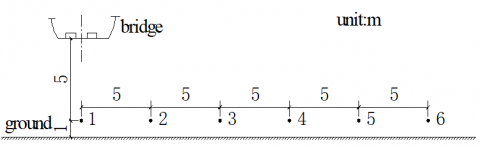Figure 4. The distribution of midspan field points

The structural noises of bridges are mainly of low frequency, which can attenuate greatly when the prevailing A-weighting evaluation index is used. Therefore, to ensure the accuracy of structural noise evaluation, the unweighted linear sound pressure level is adopted in this paper. Figure 5 is the 1/3 octave spectra of the linear sound pressure levels at 6 field points.

## 5.png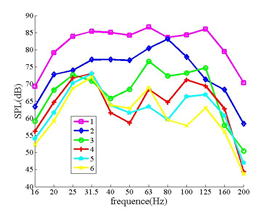Figure 5. The frequency spectrum of the sound pressure level at the field points

It can be seen from Fig.5 that field points which are further from the bridge have lower level of maximum sound pressure, and that the peak frequency of the structural noises in the channel beam is between 31.5Hz and 80Hz. Figure 5 also shows that most of the peak frequencies are concentrated in 63Hz, which fits well with the peak frequency in document (Gao et al., 2005). The reason for this phenomenon is possibly that the peak frequency of channel beam structural vibration is equal to 63Hz. Moreover, by observing the modal feature of the channel beam, we find that the vibration modes’ frequencies are more concentrated in 63Hz. If most of the rail train’s excitation frequencies are also 63Hz, it will easily cause structural resonance.

4. The structural-acoustic sensitivity analysis of the channel beam

The structural-acoustic sensitivity of the channel beam is the degree to which the sound pressure at the sound field points is sensitive to the size parameters of the channel beam. The degree of sensitivity reflects how the sound pressure change in value with the change of the structural size parameter. Positive sensitivity degree means that the sound pressure is positively related to the value of size parameter, i.e. the larger size of channel beam means less radiation noises. The inverse is also true. In this paper, the design variables include the floor slab thickness, web thickness and the thicknesses of left and right flanges. The target function is the sound pressure level at the field point 1, and the perturbation is 5%. With them, we calculate the degree to which the target function is sensitive to the design variables, and draw the calculation results in maps in Figure 6-8.

It can be seen from the figures that all the sound pressure levels at the field point 1 are positive and the largest, which indicates that increasing the thickness of the floor slab is beneficial to reducing the sound pressure level and noises at the field point. For the thin floor slab that is the direct holder of vehicle loads, if it is thickened, the bridge will become stiffer against deformation, and the structural vibration and noises will drop as well.

## 6.png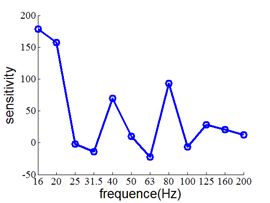Figure 6. The sensitivity of the sound pressure level at the field point 1 to the floor slab thickness

## 7.png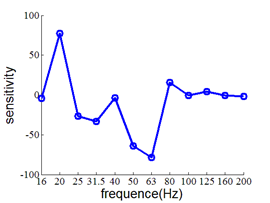Figure 7. The sensitivity of the sound pressure level to the web thickness

## 8.png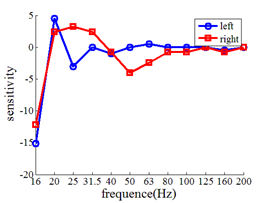Figure 8. The sensitivity of the sound pressure level to the thickness of the left and right flange

Basically none of the sensitivities of the sound pressure levels at the field point 1 to web thickness are over 80Hz, which means that thickening the web has little influence on the sound pressure level of field points whose frequency is over 80Hz. By combining Figure 5 and our calculation results, the sound pressure level at the field points near the peak frequency of 63Hz is negatively sensitive to the web thickness. This phenomenon reflects the negative effect of web thickening on denoising as with lifting the maximum sound pressure level of field points.

The sensitivities of the sound pressure levels at the field point 1 to the thickness of the left and right flanges are insignificant in the whole research period, indicating the little effect of flange thickness to the sound pressure level at field points.

5. Conclusions

By applying the vehicle-track coupled dynamics theory to a simply-supported channel beam (span: 30m), the acoustic radiation characteristics of the vehicle-loading channel beam are analyzed by using the finite element method and the indirect boundary element method. Finally, be means of finite difference method, the structural-acoustic sensitivity of the channel beam is calculated. The influence of the floor slab thickness, web thickness and the thicknesses of left and right flanges on the sound pressure levels at field points is analyzed. We draw the following conclusions:

1) the peak frequency of channel beam noises falls in the range of 31.5 Hz - 80 Hz.

2) thickening the floor slab is helpful to reduce the sound pressure level at field points, plus with obvious denoising effect.

3) thickening the web will increase the maximum sound pressure level of the sound field points in the channel beam, but the effect is little for those sound pressure levels with the frequency of over 80Hz.

Acknowledgment

This work reported here was supported by the Jiangxi science and Technology Fund Project (20181BBG70005). And by the Science and technology project of Jiangxi Provincial Department of transportation (2016H0013 and 2018H0010).

References

Bewes O. G., Thompson D. J., Jones C. J. (2006). Calculation of noise from railway bridges and viaducts: Experimental validation of a rapid calculation model. Journal of Sound and Vibration, Vol. 295, No. 24, pp. 933-943. https://doi.org/10.1016/j.jsv.2005.12.016

Chen Y. J., Ju S. H., Ni S. H. (2007). Prediction methodology for ground vibration induced by passing trains on bridge structures. Journal of Sound and Vibration, Vol. 32, No. 7, pp. 806-820. https://doi.org/10.1016/j.jsv.2006.12.010

Gao C. W., Tang Y. R., Yu H. (2005). Study on the vibration of rail transit bridge based on moving load passing bridge. China Railway Science, Vol. 26, No. 2, pp. 73-76

Janssens M. H. A., Thompson D. J. (1996). A calculation model for the noise from steel railway bridges. Journal of Sound and Vibration, Vol. 193, No. 23, pp. 295-305. https://doi.org/10.1006/jsvi.1996.0270

Lei X. Y. (2015). High Speed Railway Track Dynamics: Model, Algorithm and Application. Science Press, Beijing, pp. 85-94.

Li Q., Xu Y. L., Wu D. J. (2012). Concrete bridge-borne low-frequency noise based on rain-track-bridge dynamic interaction. Journal of Sound and Vibration, Vol. 331, No. 10, pp. 2457-2470. https://doi.org/10.1016/j.jsv.2011.12.031

Li X., Liu Q., Pei S., Song L., Zhang X. (2015). Structure-borne noise of railway composite bridge: Numerical simulation and experimental validation. Journal of Sound and Vibration, Vol. 353, No. 111, pp. 378-394. https://doi.org/10.1016/j.jsv.2015.05.030

Li X., Zhang X., Liu Q., Zhang Z., Li Y. (2013). Experimental study on structure-borne noise of railway 32 m simply-supported concrete box-girder. China Railway Science, Vol. 34, No. 3, pp. 20-26. https://doi.org/10.3969/j.issn.1001-4632.2013.03.04

Ngai K. W., Ng C. F. (2002). Structure-borne noise and vibration of concrete box structure and rail viaduct. Journal of Sound and Vibration. Vol. 255, No. 2, pp. 281-297. https://doi.org/10.1006/jsvi.2001.4155

Stuber C. (1975). Air and structure-borne noise of railways. Journal of Sound and Vibration, Vol. 43, No. 2, pp. 281-289. https://doi.org/10.1016/0022-460X(75)90327-2

Wu T. X., Liu J. H. (2012). Sound emission comparisons between the box-section and U-section concrete viaducts for elevated railway. Noise Control Engineering Journal, Vol. 60, No. 4, pp. 450-457. https://doi.org/10.3397/1.3701023

Zhai W. M. (2007). Vehicle-Track Coupled Dynamics (Third Edition). Science Press, Beijing, pp. 78-82.

Zhang H., Xie X., Jiang J. Q. (2015). Assessment on transient sound radiation of a vibrating steel bridge due to traffic loading. Journal of Sound and Vibration, Vol. 336, No. 10, pp. 132-149. https://doi.org/10.1016/j.jsv.2014.10.006

Zhang X., Li X., Hao H., Wang D., Li Y. (2015). A case study of interior low-frequency noise from box-shaped bridge girders induced by running trains: Its mechanism, prediction and countermeasures. Journal of Sound and Vibration, Vol. 367, No. 12, pp. 129-144. https://doi.org/10.1016/j.jsv.2016.01.004